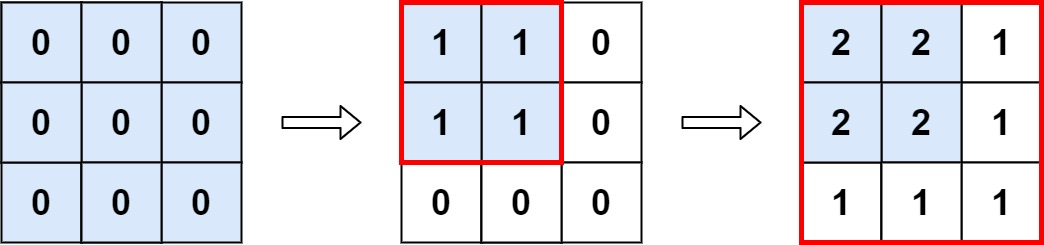You are given an `m x n` matrix `M` initialized with all `0`'s and an array of operations `ops`, where `ops[i] = [ai, bi]` means `M[x][y]` should be incremented by one for all `0 <= x < ai` and `0 <= y < bi`.

Count and return the number of maximum integers in the matrix after performing all the operations.

Example 1:```Input: m = 3, n = 3, ops = [[2,2],[3,3]]
Output: 4
Explanation: The maximum integer in M is 2, and there are four of it in M. So return 4.
```

Example 2:

```Input: m = 3, n = 3, ops = [[2,2],[3,3],[3,3],[3,3],[2,2],[3,3],[3,3],[3,3],[2,2],[3,3],[3,3],[3,3]]
Output: 4
```

Example 3:

```Input: m = 3, n = 3, ops = []
Output: 9
```

Constraints:

• `1 <= m, n <= 4 * 104`
• `1 <= ops.length <= 104`
• `ops[i].length == 2`
• `1 <= ai <= m`
• `1 <= bi <= n`

``````struct Solution;

impl Solution {
fn max_count(mut m: i32, mut n: i32, ops: Vec<Vec<i32>>) -> i32 {
for op in ops {
m = i32::min(op, m);
n = i32::min(op, n);
}
m * n
}
}

#[test]
fn test() {
let m = 3;
let n = 3;
let ops: Vec<Vec<i32>> = vec_vec_i32![[2, 2], [3, 3]];
let res = 4;
assert_eq!(Solution::max_count(m, n, ops), res);
}
``````